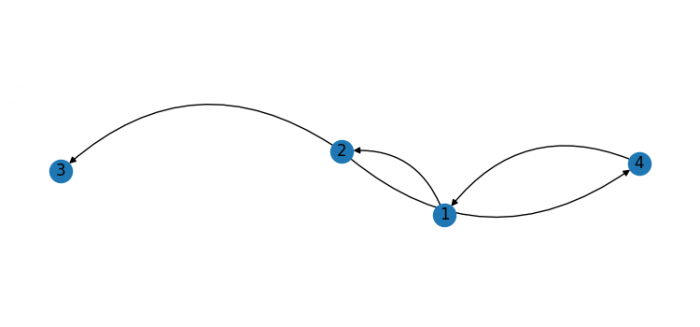# Creating curved edges with NetworkX in Python3 (Matplotlib)

PythonServer Side ProgrammingProgramming

To create curved edges with NetworkX in Python3, we can use connectionstyle="arc3, rad=0.4".

## Steps

• Set the figure size and adjust the padding between and around the subplots.
• Initialize a graph with edges, name, and graph attributes.
• Add nodes to the created graph.
• Add edges from one node to another.
• Draw the graph G with Matplotlib, with connectionstyle="arc3, rad=0.4".
• To display the figure, use show() method.

## Example

import matplotlib.pylab as plt
import networkx as nx

plt.rcParams["figure.figsize"] = [7.50, 3.50]
plt.rcParams["figure.autolayout"] = True

G = nx.DiGraph()

pos = nx.spring_layout(G)
plt.show()